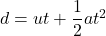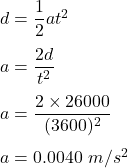## A bus started from Kathmandu and reached khanikhola,26km far from Kathmandu, in one hour. if the bus had uniform acceleration, calculate the

Question

A bus started from Kathmandu and reached khanikhola,26km far from Kathmandu, in one hour. if the bus had uniform acceleration, calculate the final velocity of the bus and acceleration. ​

in progress 0
5 months 2021-08-04T12:54:55+00:00 1 Answers 75 views 0

a = 0.0040 m/s², v = 14.4 m/s.

Step-by-step explanation:

Given that,

The distance between Kathmandu and Khanikhola, d = 26 km = 26000 m

Time, t = 1 hour = 3600 seconds

Let a is the acceleration of the bus. Using second equation of motion,Where

u is the initial speed of the bus, u = 0

So,Now using first equation of motion.

Final velocity, v = u +at

So,

v = 0+0.0040(3600)

v = 14.4 m/s

Hence, this is the required solution.